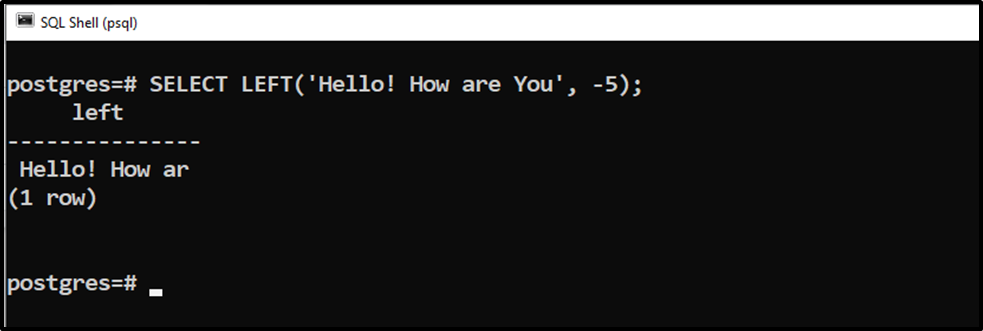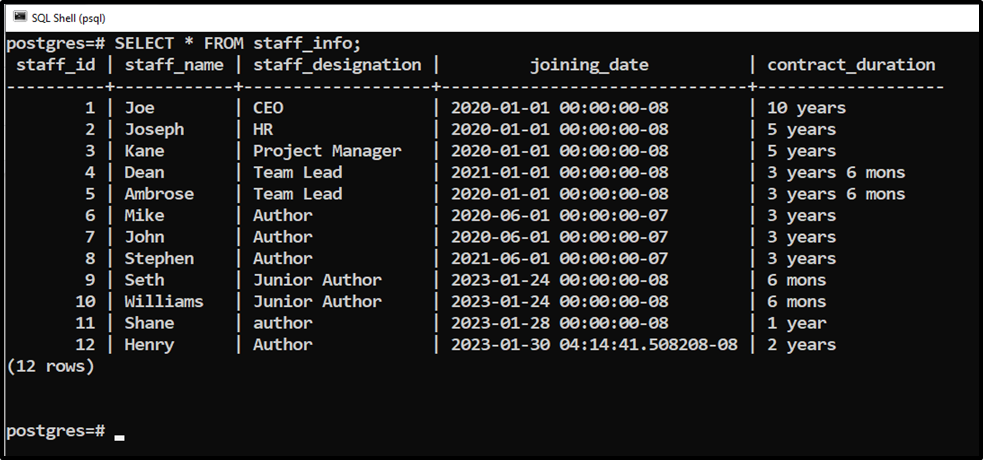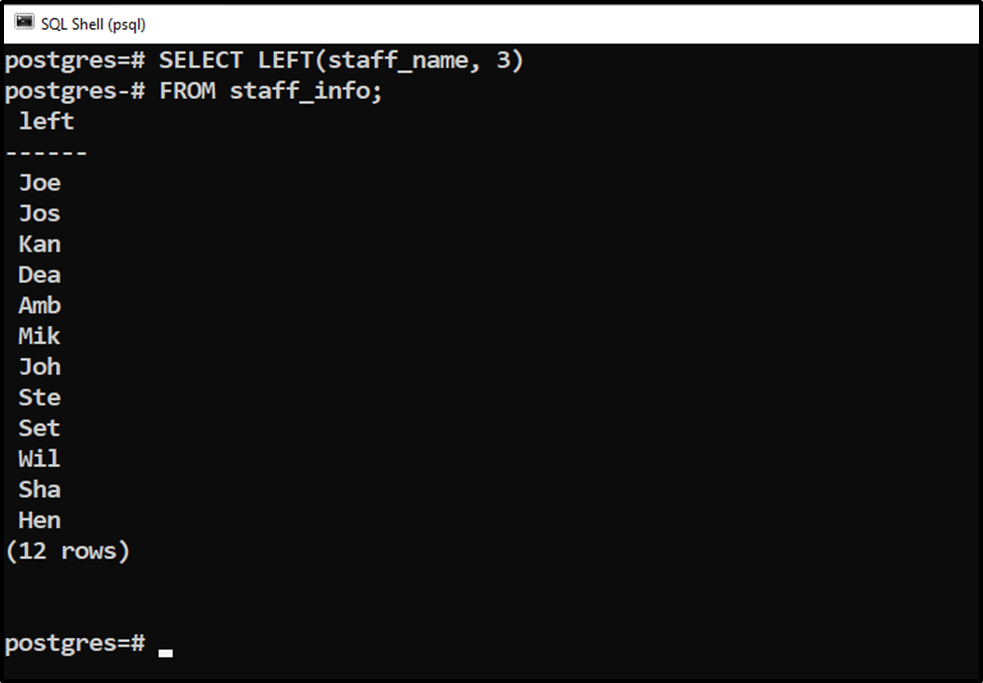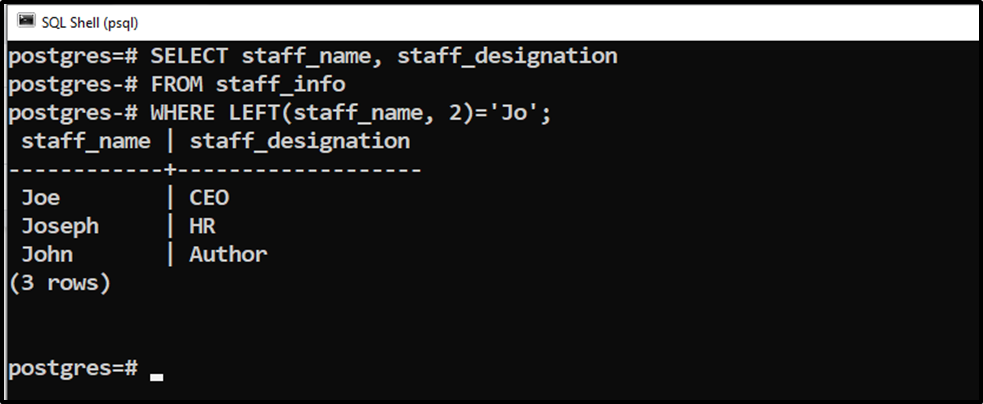##### PostgreSQL LEFT() Function: Extract Characters From the Left of a String

PostgreSQL facilitates us with numerous string functions, such as CONCAT() function, LENGTH() function, INITCAP(), etc. The LEFT() function is also a string function that is used to extract the characters from the left of the targeted string. It can extract the “n” number of characters from a string, where n can be a positive or negative value.

This postgres blog demonstrates how to use the LEFT() function in Postgres using various practical examples.

How to Use LEFT() Function in PostgreSQL?

The LEFT() function takes a string and the number of characters to extract as arguments and retrieves the extracted/modified string:

`LEFT(string, n);`

The “n” represents the number of characters extracted from a string's left. It can be positive or negative. If the value of “n” is negative, then all the string characters will be extracted except the ‘n’ rightmost characters.

Example 1: Using the LEFT() Function With a Positive Value of n

In the following query, we will extract the five characters from the leftmost of the given string using the LEFT() function:

`SELECT LEFT('Hello! How are You', 5);`The output signifies that the LEFT() function retrieves the five characters from the left of the given string.

Example 2: Using the LEFT() Function With a Negative Value of n

The following statement demonstrates how the LEFT() function work if the “n” parameter has a negative value:

`SELECT LEFT('Hello! How are You', -5);`The output indicates that the LEFT() function retrieves all the characters from a string except the five rightmost characters.

Example 3: Using the LEFT() Function on Tables Data

We have created a sample table named “staff_info” with the following data:Let’s learn how to extract the first three characters of the employee's name using the LEFT() function:

```SELECT LEFT(staff_name, 3)
FROM staff_info;```The output demonstrates that the LEFT() function retrieves the first three characters of all the employees’ names.

Example 4: Extracting Specific Records Via the LEFT() Function

Now we will utilize the LEFT() function to extract all those employees whose name starts with “Jo”:

```SELECT staff_name, staff_designation
FROM staff_info
WHERE LEFT(staff_name, 2)='Jo';```The output demonstrates that the LEFT() function retrieves the designation of all those staff members whose names start with “Jo”.

Conclusion

The LEFT() function takes a string and the number of characters to extract as arguments and retrieves the extracted/modified string. It can extract the “n” number of characters from a string, where n can be a positive or negative value. If the value of “n” is negative, then all the string characters will be extracted except the “n” leftmost characters. This post explained the usage of the LEFT() function using practical examples.